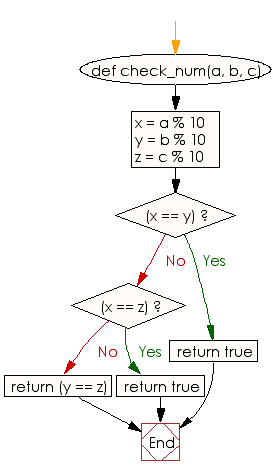﻿ Ruby Basic exercises: Check three given integers and return true if two or more of them have the same rightmost digit - w3resource# Ruby Basic Exercises: Check three given integers and return true if two or more of them have the same rightmost digit

## Ruby Basic: Exercise-48 with Solution

Write a Ruby program to check three given integers and return true if two or more of them have the same rightmost digit.

Ruby Code:

``````def check_num(a, b, c)
x = a % 10
y = b % 10
z = c % 10
if(x == y)
return true
end
if(x == z)
return true
end
return (y == z)
end
print check_num(9, 12, 22),"\n"
print check_num(112, 202, 52),"\n"
print check_num(102, 203, 405)
``````

Output:

```true
true
false
```

Flowchart:Ruby Code Editor: